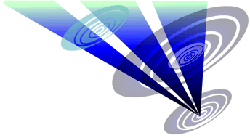Reconstruction of the 2019 Stanton St. Bernard formation 1.Draw a circle. Draw and extend the horizontal and vertical centerlines. 2.Construct the inscribed hexagon (regular 6-sided polygon) of circle 1, pointing up. 3.Draw the six rays of circle 1, to the angular points of hexagon 2. Number these rays 1 - 6 clockwise, starting at the top. 4.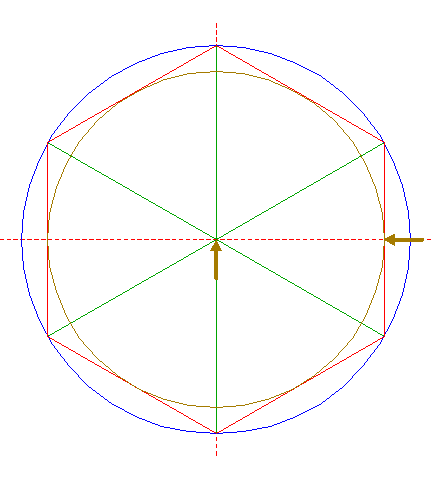Construct the inscribed circle of hexagon 2. 5.Construct two inscribed equilateral triangles of circle 1, one pointing up, one pointing down. 6.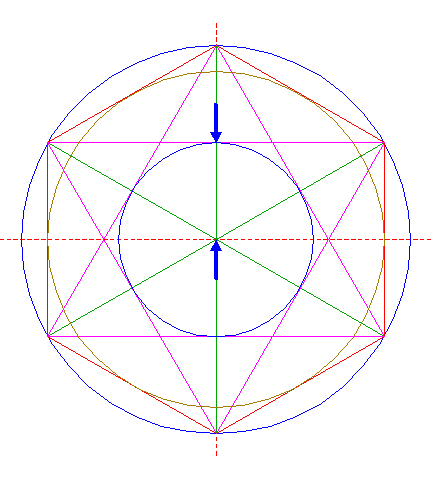Construct the inscribed circle of the hexagon enclosed by triangles 5. 7.Construct two inscribed equilateral triangles of circle 6, one pointing up, one pointing down. 8.Construct the inscribed circle of the hexagon enclosed by triangles 7. 9.Construct two inscribed equilateral triangles of circle 8, one pointing up, one pointing down. 10.Construct the inscribed circle of the hexagon enclosed by triangles 9. 11.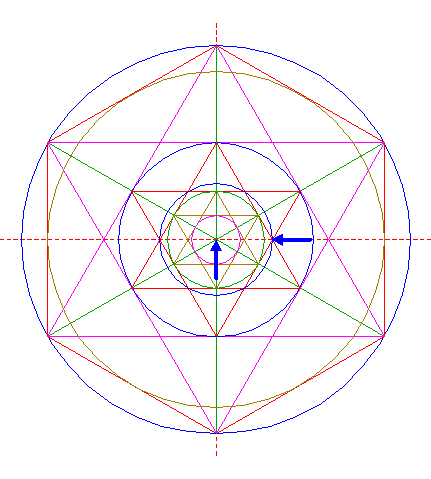Construct a circle (concentric to circle 1) passing through the mutual intersections of triangles 7. 12.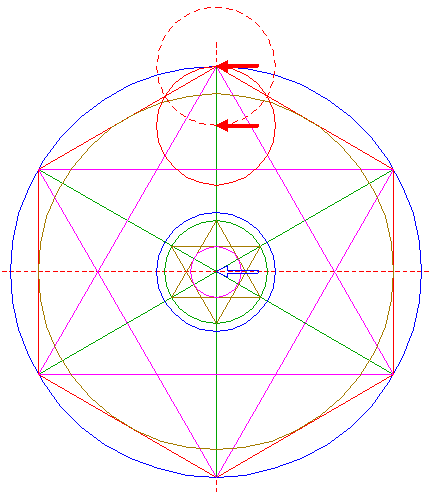Copy circle 11 to the top of hexagon 2, and move this circle (copy and delete original) to its own lower intersection with the vertical centerline. 13.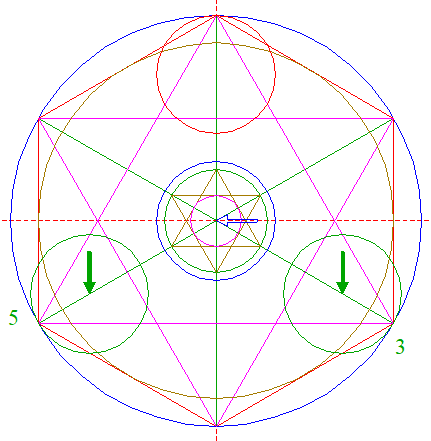Copy circle 11 two times, to the corresponding positions on rays 3 nrs. 3 and 5. 14.Construct a circle centered at the intersection of circle 4 and ray 3 nr. 2, passing through the righthand intersection of circle 4 and the upper horizontal side of triangles 5. 15.Copy circle 14 to the intersection of circle 10 and ray 3 nr. 2. 16.Construct a circle concentric to circle 1, tangent to circle 15 at the upper righthand side. 17.Copy circle 16 to the top of hexagon 2, and move this circle to its own lower intersection with the vertical centerline. 18.Copy circle 16 five times, to the corresponding positions on the other rays 3 (nrs. 2, 3, 4, 5, and 6). 19.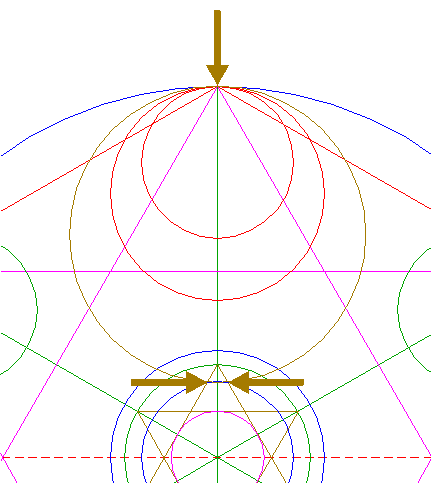Construct a circle passing through the top of hexagon 2, and passing through the upper two intersections of circle 16 and triangles 9. 20.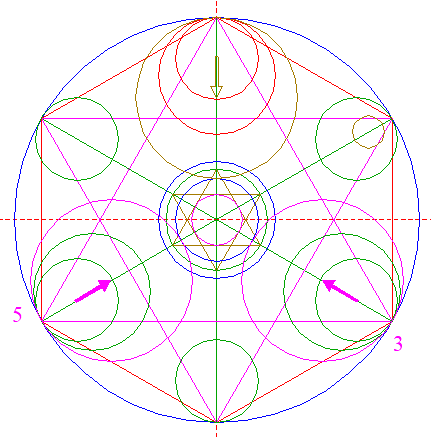Copy circle 19 two times, to the corresponding positions on rays 3 nrs. 3 and 5. 21.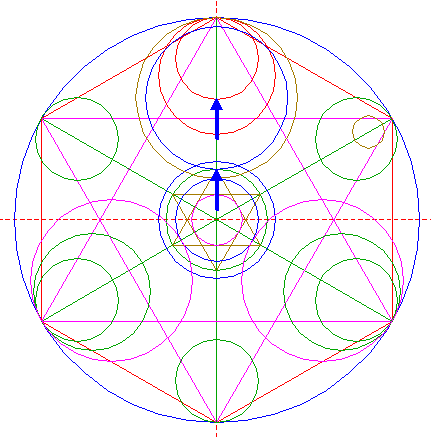Construct a circle concentric to circle 19, tangent to circle 8 at the upper side. 22.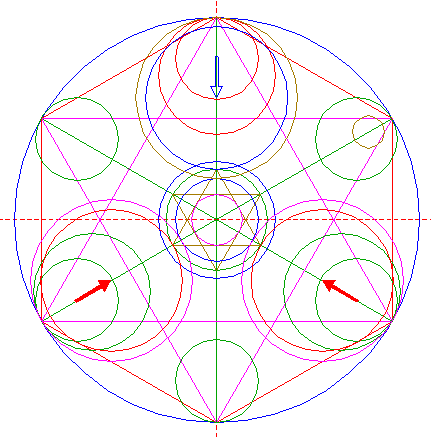Copy circle 21 two times, to the centers of circles 20. 23.Construct a circle centered at the lower lefthand intersection of circle 14 and ray 3 nr. 2, passing through the other intersection. 24.Copy circle 23 to the center of circle 1. 25.Construct a circle centered at the intersection of the righthand side of hexagon 2 and the horizontal centerline, tangent to the nearest sides of triangles 5. 26.Construct a circle concentric to circle 1, tangent to circle 25 at the righthand side. 27.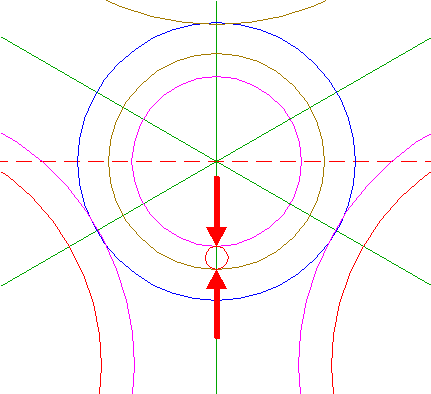Construct a "two-points" circle (defined by the two end-points of a centerline) between the lower intersections of circles 10 and 24 with the vertical centerline. 28.Copy circle 27 two times, to the centers of circle 1 and the lower circle 18. 29.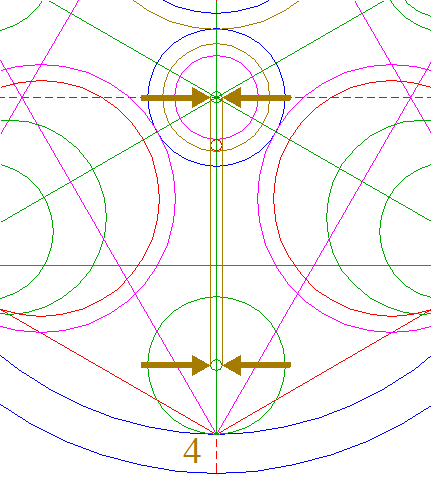Construct two lines, parallel to ray 3 nr. 4, tangent to circles 28 at both sides. 30.Repeat steps 28 and 29 two times, with respect to rays 3 nrs. 2 and 6. 31.Circles 1, 10, 12, 13, 16, 17, 18, 19, 20, 21, 22, 24, and 26, and lines 29 and 30, are used for the final reconstruction. 32.Remove all parts not visible within the formation itself. 33.Colour all areas corresponding to standing... 34.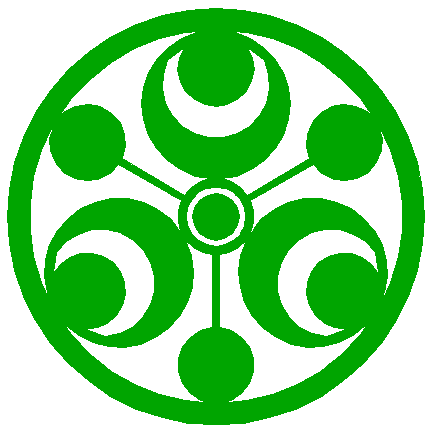...or to flattened crop, and finish the reconstruction of the 2019 Stanton St. Bernard formation. 35.The final result, matched with the aerial image.